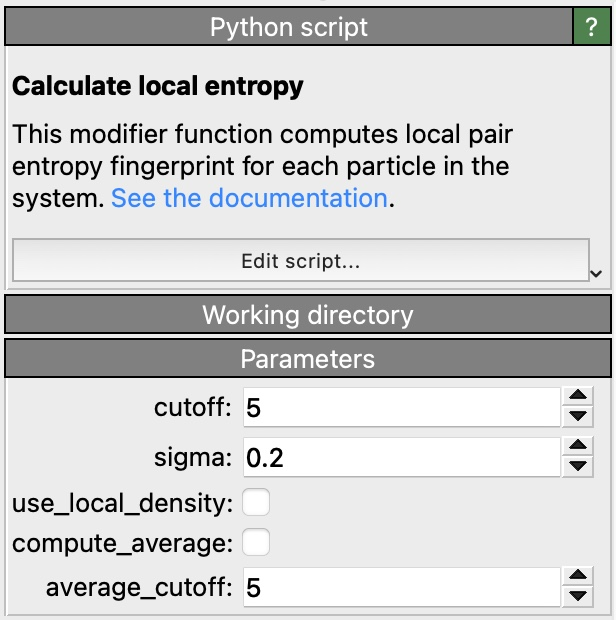$$\renewcommand\AA{\text{Å}}$$

# Calculate local entropy pro

This is a Python-based modifier function computing the local pair entropy fingerprint of each particle as described in:

P.M. Piaggi and M. Parrinello,
Entropy based fingerprint for local crystalline order,
J. Chem. Phys. 147, 114112 (2017)

The modifier function stores the computed pair entropy values, which are always negative, in the output particle property Entropy. Lower entropy values correspond to more ordered structural environments. The modifier function does not take into account the types of the input particles; it assumes the model is a single-component system.

The calculation algorithm follows closely the C++ implementation of the compute entropy/atom command of the LAMMPS MD code. The meaning of the input parameters of the modifier is the same as for the LAMMPS command.

## ParametersCutoff

Cutoff distance for the $$g(r)$$ calculation.

Sigma

Width of Gaussians used in the $$g(r)$$ smoothing.

Use local density

Use the local density around each atom to normalize the $$g(r)$$.

Compute average

Average the pair entropy values over neighbors.

Average cutoff

Cutoff distance for the averaging over neighbors.